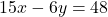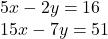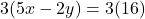Question

Consider the system of equations

5x-2y=16
15x-7y=51

Multiply the first equation by some constant, so that in the resulting equation, the coefficient of matches the coefficient of in the second equation.

Enter the entire equation that results from multiply

in progress 0
5 months 2021-08-09T20:21:23+00:00 2 Answers 8 views 0Step-by-step explanation:

So we have the system of equations:And we want to multiply the first equation by some constant such that the resulting equation matches the coefficient of [x] in the second equation.

We can see that the coefficient of x in the second equation is 15.

So, to match 15, we must multiply the first equation by 3. So:Multiply:And we’re done!

Step-by-step explanation:

-15x + 6y = -48

15x – 7x = 51

-x = 3

x = -3

5(-3) – 2y = 16

-15 – 2y = 16

-2y = 31

y = -15.5

(-3, -15.5)